[MyHosting.com]   [KO4BB Home Page]   [Manuals Home Page]   [KO4BB Wiki]

# Differences

This shows you the differences between two versions of the page.

 — precision_timing:outliers_how_to_identify_and_eliminate_them [2013/01/08 19:00] (current) Line 1: Line 1: + ====== Outliers, how to Identify and Eliminate them ====== + + ---- + + From Ulrich Bangert, on the Time-Nuts mailing list + + Discarding outliers in two dimensions + + >  ​ + > Suppose I want to average a bunch of samples. ​ Sometimes it helps to + > discard the outliers. ​ I think that helps when there are two noise + > mechanisms, say the typical Gaussian plus sometimes some other noise + > added on.  If the other noise is rare but large, those occasional ​ + > samples can have a big influence on the average. ​ So discarding those + > outliers gives better results, for some value of "​better"​. + > + > I know how to do it in one dimension. ​ How do I do it in two + > dimensions? + > + > Hal Murray + >  ​ + + ....Well, may work... A Method of outlier search in one dimension that has worked for me very well in the last years is (it is not my idea but comes from an article covering robust statistics):​ + + First you have to understand that the usual arithmetic average and the standard deviation are measures that are NOT robust against outliers and that you need to substitute them by robust measures when you need their functionality. + + 1) Sort Data in ascending order. + + 2) Find the "​center"​ of the sorted data, i.e. the data value where 50 % of all values are greater or equal and the other 50 % are smaller or equal the specific value. This value is called the "​median"​ or "50% percentile"​. + Imagine it as a substitute for the average that is VERY robust. + + 3) Now (similar as with the standard deviation) compute the absolute values of the differences of all data points and the median. + + 4) Again order the resulting values in ascending order and find their median. + + 5) What you have now is the median deviation of the data to the original median and is a very robust measure of the width of the distribution. There is even a "​norming"​ factor (that I do not remember because I do not need it) that makes this number directly comparable to the standard deviation of (outlier free) data. 99% of all data of a Gaussian distribution are inside +/- 3 sigma, so if a data value is outside say +/- 5 median deviation, then + it is very likely a outlier. + + However, what you really want is an outlier free average value. The median itself is a single data value containing all the noise that you want to average out. For this purpose robust statistics holds a different (but similar) tool: The IQR (Inner Quartile Range). The algorithm is: + + 1) Sort data in ascending order + + 2) Find the median of the data, the 50 % percentile but in addition also find the 25% percentile and the 75 % percentile. + + 3) Now you have 4 groups (quartiles) of data, divided by the 3 percentiles. + Ignore the outer quartiles (where outliers are located) and compute the arithmetic average over the two inner two quartiles which are free of outliers if at least 50 % of all data are NOT outliers. ​ + + The IQR is a robust compromise between outlier removal and noise removal. + + For the two dimension case I would suggest the following: + + 1) For all computations keep the index of the data points with you so that a data point can be identified later. + + 2) Sort data in ascending order separate for the two dimensions. + + 3) Identify the inner quartiles separate for the two dimensions. + + 4) Now search for indices that are contained in BOTH inner quartiles, i.e. + data that has NOT been sorted out as a outlier in one of the dimensions. + + 4) Compute the arithmetic average over the data points found in 4) + + Best regards + + Ulrich

precision_timing/outliers_how_to_identify_and_eliminate_them.txt · Last modified: 2013/01/08 19:00 (external edit)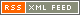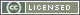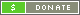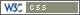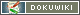##### Except as noted, this entire site Copyright © 2002-2017. KO4BB All rights reserved.